Rule 1:

If different distance is travelled in different time then,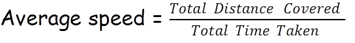Example

If a car travels 50 Km in 1 hour, another 40 Km in 2 hour and another 70 Km in 3 hour then what is average speed of car.

Sol: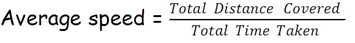Total Distance Covered = 50 + 40 + 70 = 160 Km

Total Time Taken = 1 + 2 + 3 = 6 hours.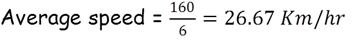Rule 2:

If equal distance is travelled at different speed.

If equal distance is travelled at the speed of A and B then,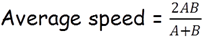Example

A boy goes to his school which is 2 Km away in 10 minutes and returns in 20 mins then what is boy’s average speed.

Sol:Let’s say A = 2/10 = 0.2 km/min

And B = 2/20 = 0.1 km/minRule 3:

If equal distance is travelled at the speed of A, B and C then,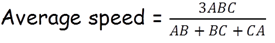Example

If a car divides its total journey in three equal parts and travels those distances at speed of 60 kmph, 40 kmph and 80 kmph then what is car’s average speed?

Sol:Let’s say A = 60, B = 40 and C = 80, then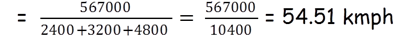Go to next page>>